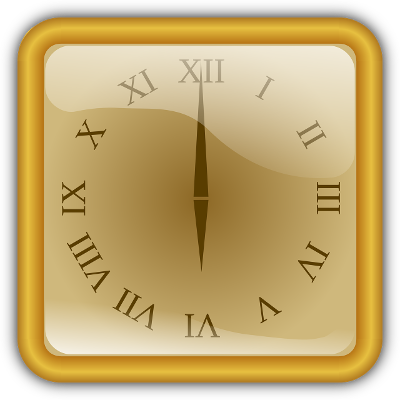# Roman Numerals Converter

Share:

Select the type of conversion, either whole number (Hindu-Arabic number) to roman numeral or roman numeral to whole number.

Enter a whole number (Hindu-Arabic number) between 1 and 3,999,999,999 (1 less than 4 billion) and click Convert.

Result:

## Using The Number to Roman Numeral Converter

1. Enter a whole number between 1 and 3,999,999,999 (1 less than 4 billions).
2. Press Convert button.
3. The equivalent roman numerals will be displayed in the result section.

## Whole Number To Roman Numeral Conversion Chart

### Generating And Printing The Whole Number To Roman Numeral Conversion Chart

1. Enter the Starting Number you desire to start the conversion chart.
2. Enter the Intrement between the numerals. Example: 1, 10, 100, etc...
3. Click the Generate Chart button to generate your desired chart.
4. Click the Print button to print the chart you have generated. This printing option allows you to print only the chart portion.

Start. Value
Increment

Number Roman
10 X
20 XX
30 XXX
40 XL
50 L
60 LX
70 LXX
80 LXXX
90 XC
100 C
110 CX
120 CXX
130 CXXX
140 CXL
150 CL
160 CLX
170 CLXX
180 CLXXX
190 CXC
200 CC
210 CCX
220 CCXX
230 CCXXX
240 CCXL
250 CCL
Number Roman
260 CCLX
270 CCLXX
280 CCLXXX
290 CCXC
300 CCC
310 CCCX
320 CCCXX
330 CCCXXX
340 CCCXL
350 CCCL
360 CCCLX
370 CCCLXX
380 CCCLXXX
390 CCCXC
400 CD
410 CDX
420 CDXX
430 CDXXX
440 CDXL
450 CDL
460 CDLX
470 CDLXX
480 CDLXXX
490 CDXC
500 D
Number Roman
510 DX
520 DXX
530 DXXX
540 DXL
550 DL
560 DLX
570 DLXX
580 DLXXX
590 DXC
600 DC
610 DCX
620 DCXX
630 DCXXX
640 DCXL
650 DCL
660 DCLX
670 DCLXX
680 DCLXXX
690 DCXC
700 DCC
710 DCCX
720 DCCXX
730 DCCXXX
740 DCCXL
750 DCCL
Number Roman
760 DCCLX
770 DCCLXX
780 DCCLXXX
790 DCCXC
800 DCCC
810 DCCCX
820 DCCCXX
830 DCCCXXX
840 DCCCXL
850 DCCCL
860 DCCCLX
870 DCCCLXX
880 DCCCLXXX
890 DCCCXC
900 CM
910 CMX
920 CMXX
930 CMXXX
940 CMXL
950 CML
960 CMLX
970 CMLXX
980 CMLXXX
990 CMXC
1000 M

## Roman Numerals

### Symbols Of Roman NumeralsThe number system followed by the ancient roman people. Conventional roman numerals are basically a combination of Latin alphabets I, V, X, L, C, D and M. This conventional system can generate roman numerals up to 4,999. The extend the roman system beyond this limit, several other systems were introduced. One among the system is Vinculum. In this system the traditional roman numeral symbol is represented by a overline (I, V, X, L, C, D and M) in order to multiply it by thousand (1,000). This can be further extended by adding double overlines (I, V, X, L, C, D and M) to multiple it by million (1,000,000).

Because, overlines and double overlines (M, M) are difficult to use in computer user interface, the alternative symbol using single and double brackets (M) and ((M)) are used in recent years.

Below is the table of conventional and extended roman numeral symbols along with the recent alternative symbols using brackets instead of overlines.

Symbol Value Alternative Symbol
I 1
V 5
X 10
L 50
C 100
D 500
M 1,000
I 1,000 (I)
V 5,000 (V)
X 10,000 (X)
L 50,000 (L)
C 100,000 (C)
D 500,000 (D)
M 1,000,000 (M)
I 1,000,000 ((I))
V 5,0000,00 ((V))
X 10,000,000 ((X))
L 50,000,000 ((L))
C 100,000,000 ((C))
D 500,000,000 ((D))
M 1,000,000,000 ((M))

### Rules Of Roman Numerals

There are few set of rules to be followed while using roman numerals. Initially, in the conventional system, the romans used only up to 3,999. So, the rules were based on the numerals up to 3,999. Later on, when more symbols are added to extend the numeral, few more rules were added on to accommodate the new symbols.

1. #### Repetition Rule

• The symbols I, X, C, M, I, I, X, C, M, I, X, C, M can be repeated in succession only up to three times. For example, 8 is represented as VIII.
• The Symbols V, L, D, V, L, D, V, L, D should not be repeated. they can be used in a number only once.

When a symbol of lesser value is in the right of a symbol of a similar value or greater value then the symbols should be added.

For example, If the number is represented as XV then the value of X and V should be added, i.e. XV = 10 + 5 = 15

3. #### Subtraction Rule

• When a symbol of lesser value is in the left side of a symbol of a greater value then the symbols should be subtracted.

Subtraction rule comes in effort only when a number cannot be generated using the addition rule.

For example, If the number is represented as IX, then the value of I should be subtracted from X, i.e. IX = 10 - 1 = 9.

• When a symbol of smaller value is placed in between two symbols of greater value, it shouls be subtracted from the symbol on the right.

It should not be added to the the symbol on its left.

For example, If a number is represented as LIX, then then the value of I should be subtracted from X, i.e. LIX = L + (X - I) = 50 + (10 - 1) = 59.

4. #### Larger Numbers

To represent numbers beyond 3,999 a single-overline or double-overline can be added to the basic conventional symbols so as to multiply the number by 1,000 or 1,000,000 respectively.

##### For Example:

X = 10 x 1,000 = 10,000 (Ten Thousand).

X = 10 x 1,000,000 = 10,000,000 (Ten Million).